Note: This is an archvied version of our old webpage. Some links might be broken. The current one can be found here.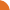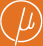Chair for Foundations of Software Reliability and Theoretical Computer Science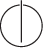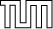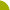Publications - On the Convergence of Newton's Method for Monotone Systems of Polynomial EquationsReference: Stefan Kiefer, Michael Luttenberger, and Javier Esparza. On the convergence of Newton's method for monotone systems of polynomial equations. In Proceedings of the 39th ACM Symposium on Theory of Computing (STOC), pages 217–226, San Diego, California, USA, 2007. ACM. Abstract: Monotone systems of polynomial equations (MSPEs) are systems of fixed-point equations X_1 = f_1(X_1, ..., X_n), ..., X_n = f_n(X_1, ..., X_n) where each f_i is a polynomial with positive real coefficients. The question of computing the least non-negative solution of a given MSPE X = f(X) arises naturally in the analysis of stochastic context-free grammars, recursive Markov chains, and probabilistic pushdown automata. While the Kleene sequence f(0), f(f(0)),... always converges to the least solution mu.f, if it exists, the number of iterations needed to compute the first i bits of mu.f may grow exponentially in i. Etessami and Yannakakis have recently adapted Newton's iterative method to MSPEs and proved that the Newton sequence converges at least as fast as the Kleene sequence and exponentially faster in many cases. They conjecture that, given an MSPE of size m, the number of Newton iterations needed to obtain i accurate bits of mu.f grows polynomially in i and m. In this paper we show that the number of iterations grows linearly in i for strongly connected MSPEs and may grow exponentially in m for general MSPEs. Suggested BibTeX entry: @inproceedings{KLE07:stoc,     address = {San Diego, California, USA},     author = {Stefan Kiefer and Michael Luttenberger and Javier Esparza},     booktitle = {Proceedings of the 39th ACM Symposium on Theory of Computing (STOC)},     pages = {217--226},     publisher = {ACM},     title = {On the Convergence of {N}ewton's Method for Monotone Systems of Polynomial Equations},     year = {2007} }